Sie sind hier: ICP » R. Hilfer » Publikationen

# 7 Induced Measure Preserving Transformations

[212.4.1] Consider a subset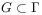with small but positive measure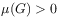of a measure preserving many body system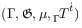. [page 213, §0]    [213.0.1] Because ofthe subset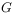becomes a probability measure space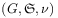with induced probability measure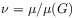and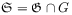being the trace of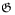in.

[213.1.1] The measure preserving continuous time evolution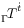is discretized by setting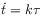(11)

with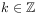and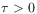the discretization time step. [213.1.2] A character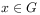is called recurrent, if there exists an integer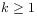such that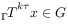. [213.1.3] If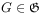and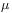is invariant under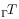, then almost every character inis recurrent by virtue of the Poincarè recurrence theorem. [213.1.4] A subsetis called recurrent, if-almost every pointis recurrent. [213.1.5] By Poincarè’s recurrence theorem the recurrence time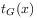of the character, defined as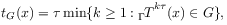(12)

is positive and finite for almost every. [213.1.6] For everylet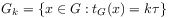(13)

denote the set of characters with recurrence time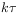. [213.1.7] Then the number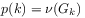(14)

is the probability to find a recurrence time. [213.1.8] The numbers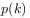define a discrete probability density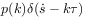on the arithmetic progression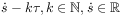. [213.1.9] Every probability measure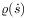on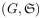at time instant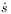is then defined on the same arithmetic progression through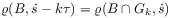(15)

for all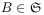and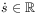. [213.1.10] The induced time evolution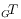on the subsetis defined for everyas the average [1, 21]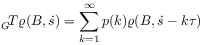(16)

where. [213.1.11] For characters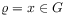, one recovers the first step in the discretized microscopic time evolution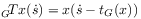as expected. [213.1.12] For mixed states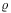this formula allows the transition from the microscopic to the macroscopic time evolution. [213.1.13] It assigns an averaged translation to the first step in the induced time evolution of mixed states.